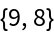#Function Repository Resource:

# DropLargest

Drop the largest values from a list

Contributed by: Sander Huisman  |  SHuisman
 ResourceFunction["DropLargest"][list,n] drops the largest n numbers from list. ResourceFunction["DropLargest"][n] represents an operator form of ResourceFunction["DropLargest"] that can be applied to an expression.

## Examples

### Basic Examples (2)

Drop the largest two values:

 In:=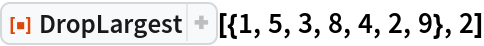Out=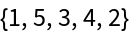Use the operator form, by first creating an operator op:

 In:=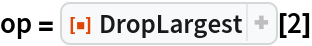Out=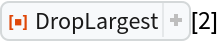Apply the operator:

 In:=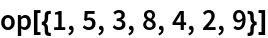Out=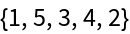### Scope (2)

When n=0, nothing is deleted:

 In:=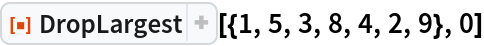Out=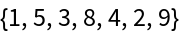A negative number deletes the smallest n numbers:

 In:=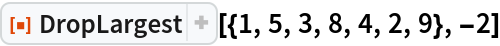Out=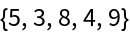### Properties and Relations (2)

If n is larger than the length of the list, an empty list is returned:

 In:=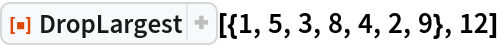Out=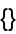DropLargest is complementary with TakeLargest:

 In:=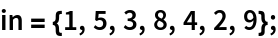In:=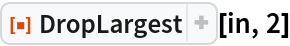Out=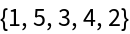In:=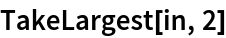Out=